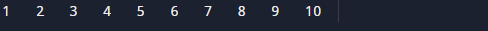C Program To Print First 10 Natural Numbers

In this tutorial you will learn about the C Program To Print First 10 Natural Numbers and its application with practical example.

In this tutorial, we will learn to create a C program that will display First 10 Natural numbers       using C programming.

Prerequisites

Before starting with this tutorial we assume that you are best aware of the following C programming topics:

• C Operators
• C loop statements

What Is Natural  Number?

Natural numbers As such, it is a whole, non-negative are all number 1, 2, 3, 4,5,6,7,8,9,10… They are usually counting  number and they will continue to infinity.while Whole number are all natural numbers including 0.

ExampleC program to display first 10 natural numbers.

In this program we will print  starting 10  natural integer values. Using For loop.
C Program to Print Natural Numbers from 1 to 10 using for Loop.

outputIn the above program, we have first declared and initialized a set variables required in the program.

• j= for iteration of loop.

In this program we will need only one variable for traversing first 10 natural number.

Within this Program to display 1 to 10 natural number.

• The first we will create  an integer variable j.
• then, we use For Loop to iterate between j=1 to j<=10 and
• Within the For loop, we print the value of j .
• In our  C Programming example, shows  value from 1- 10.that’s all fro this post thank-you.

In this tutorial we have learn about the C Program To Print First 10 Natural Numbers and its application with practical example. I hope you will like this tutorial.